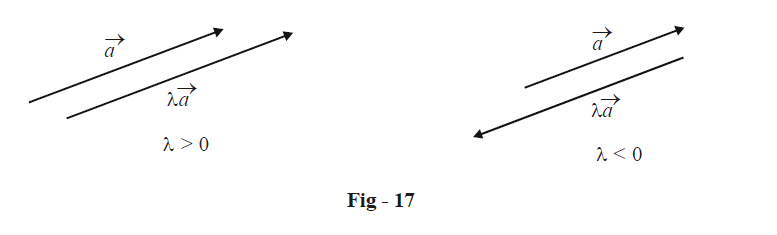# Scalar Vector Multiplication And Linear Combinations Of Vectors

Go back to  'Vectors and 3-D Geometry'

(C) MULTIPLICATION OF A VECTOR BY A SCALAR

Intuitively, we can expect that if we multiply a vector $$\vec a$$ by some scalar $$\lambda$$, the support of the vector will not change; only its magnitude and / or its sense will. Specifically, if $$\lambda$$ is positive, the vector will have the same direction; only its length will get scaled according to the magnitude of $$\lambda$$. If $$\lambda$$ is negative, the direction of the product vector will be opposite to that of the original vector; the length of the product vector will depend on the magnitude of $$\lambda$$ .Note that for any vector $$\vec a$$, if we denote the unit vector along $$\vec a$$ by $$\hat a$$, we have

$\boxed{\vec a = \left| {\,\vec a\,} \right|\hat a}$

Put in words, if we multiply the unit vector along a vector  $$\vec a$$ by its magnitude, we obtain that vector itself. Put in a slightly different way, we have

$\hat a = \frac{{\vec a}}{{\left| {\vec a} \right|}}$

i.e, if we divide a vector by its magnitude, we obtain the unit vector along that vector’s direction.

Another very important result that follows from this discussion is that two vectors $$\vec a\,\,{\text{and}}\,\,\vec b$$ are collinear if and only if there exists some  $$\lambda \in \mathbb{R}$$ such that

$\boxed{\vec a = \lambda \vec b}{\quad \mathbf{Collinear}}{\text{ }}{\mathbf{vectors}}$

i.e, two vectors are collinear if one can be obtained from the other simply by multiplying the latter with a scalar.

This fact can be stated in another way : consider two non-collinear vectors $$\vec a\,\,{\text{and}}\,\,\vec b$$  . If for some $$\lambda ,\mu \in \mathbb{R}$$, the relation

$\lambda \vec a + \mu \vec b = \vec 0\quad\quad\quad...{\text{ }}\left( 1 \right)$

is satisfied, then $$\lambda \,and\,\mu$$  must be zero. This is because (1) can be written as

$\vec a = \left( { - \frac{\mu }{\lambda }} \right)\vec b$

which would imply that $$\vec a$$  is a scalar multiple of $$\vec b$$ ,i.e., $$\vec a\,\,{\text{and}}\,\,\vec b$$ are collinear, contradicting our initial supposition that $$\vec a\,\,{\text{and}}\,\,\vec b$$ are non-collinear.

In subsequent discussions, we’ll be talking a lot about linear combinations of vectors. Let us see what we mean by this. Consider n arbitrary vectors $${\vec a_1},{\vec a_2}......{\vec a_n}.$$ A linear combination of these n vectors is a vector $$\vec r$$ such that

$\vec r = {\lambda _1}{\vec a_1} + {\lambda _2}{\vec a_2} + ...... + {\lambda _n}{\vec a_n}\quad\quad\quad...{\text{ }}\left( 2 \right)$

where $${\lambda _1},{\lambda _2}....{\lambda _n} \in \mathbb{R}$$ are arbitrary scalars. Any sort of combination of the form in (2) will be termed a linear combination.

Thus, using the terminology of linear combinations, we can restate the result we obtained earlier: for any two non-zero and non-collinear vectors  $$\vec a\,\,{\text{and}}\,\,\vec b$$, if their linear combination is zero, then both the scalars in the linear combination must be zero.

We now come to a very important concept.

Vectors
grade 11 | Questions Set 1
Vectors